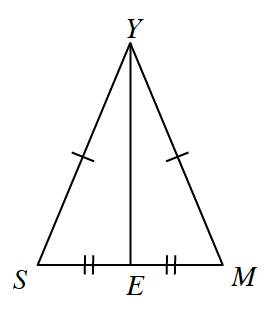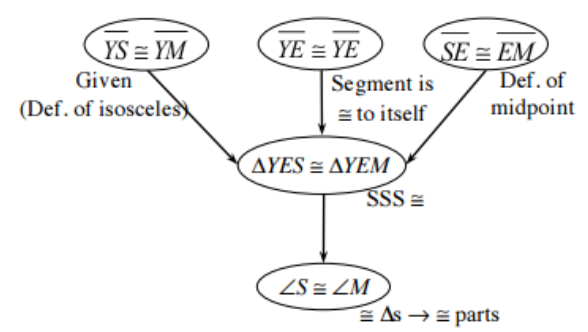### Home > INT2 > Chapter 2 > Lesson 2.1.2 > Problem2-17

2-17.

In an isosceles triangle, the two angles opposite the congruent sides are called the base angles. You may have learned in a previous course that the base angles of an isosceles triangle are always congruent. Now you will prove it! In the diagram at right, $∆\ SYM$ is an isosceles triangle, and point $E$ is the midpoint of $\overline{SM}$ .1. ​Make a flowchart to prove that the base angles of $∆\ SYM$ are congruent, that is, prove that $∠S ≅ ∠M$.1. Would your proof work for any isosceles triangle? State your findings as an if-then statement and add it to your Theorem Graphic Organizer.

Since we found this to be true, formulate an if-then statement to conclude information from the flowchart.